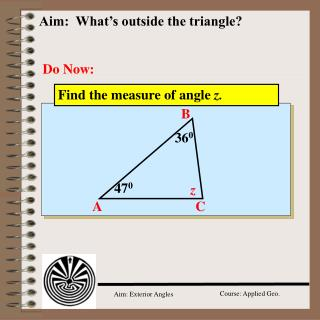Download PresentationAim: What’s outside the triangle?Aim: What’s outside the triangle? - PowerPoint PPT Presentation

Download PresentationAim: What’s outside the triangle?
An Image/Link below is provided (as is) to download presentation

Download Policy: Content on the Website is provided to you AS IS for your information and personal use and may not be sold / licensed / shared on other websites without getting consent from its author. While downloading, if for some reason you are not able to download a presentation, the publisher may have deleted the file from their server.

- - - - - - - - - - - - - - - - - - - - - - - - - - - E N D - - - - - - - - - - - - - - - - - - - - - - - - - - -
Presentation Transcript

1. 360 470 Aim: What’s outside the triangle? Do Now: Find the measure of angle z. B z C A

2. 360 470 830 Find the measure of angle z and x. B 47 + 36 = 83 The sum of the measure of the angles of a triangle is 1800. 830 x z 970 C A D 47 + 36 + z = 180 83 + z = 180 z = 97 ACB and BCD are supplementary 97+x = 180 x = 83

3. remote interior angles exterior angle B 360 1440 470 970 A E z 1440 47 + 97 = 144 C ABC and CDE are supplementary 36 + z = 180 z = 144

4. remote interior angles B exterior angle 360 1330 470 970 A z C E 36 + 97 = 133 CAB and BAE are supplementary 47 + z = 180 z = 133

5. B Exterior Angles x y w A C An exterior angle of a triangle is an angle formed by a side of the triangle and the extension of another side of the triangle. wis an exterior angle of triangle ABC x& y are nonadjacent interior angles often called remote interior angles The measure of an exterior angle of a triangle is equal to the sum of the measures of the two nonadjacent interior angles. w = x + y Exterior Angle Theorem

6. 50o 130o Find the value of variable s 130 128.50 Exterior Angle Theorem s0 s0 + 130 = 128.50 s0 = 115.50 Find the value of variable s 80o so 50o Isosceles triangle Base angles of an isosceles triangle are congruent.

7. Model Problems = 70 105 = 70 35 F mQTS = mx = 70 alternate interior s 105 = 70 + z Exterior Angle Theorem 35 = z

8. 5 4 2 3 1 Model Problems Explain how you find m3 if m5 = 130 and m4 = 70. = 130o = 70o m5 is an exterior angle of a triangle. Its measure equals the sum of m3 + m4, the remote interior angles of the triangle. Given that m5 = 1300 and m4 = 700, m3 must be 600, the difference between m5 and m4.

9. Model Problems C 80o 2x + 30 130o 50o 50o xo xo D A B Isosceles triangle mDAC + mCAB = 180 2x + 30 + x = 180 3x + 30 = 180 3x= 150 x= 50 Base angles of an isosceles triangle are congruent.

10. = 35 = 85 1200 The measure of an exterior angle of a triangle is 1200. If the measure of one of the remote angles is 200 less than three times the other remote angle, find the measure of the two remote angles. Exterior Angle Theorem 3x - 20 1200 x x = smaller of two remote angles 3x - 20 = larger of two 3x – 20 + x = 120 4x – 20 = 120 4x = 140 x = 35